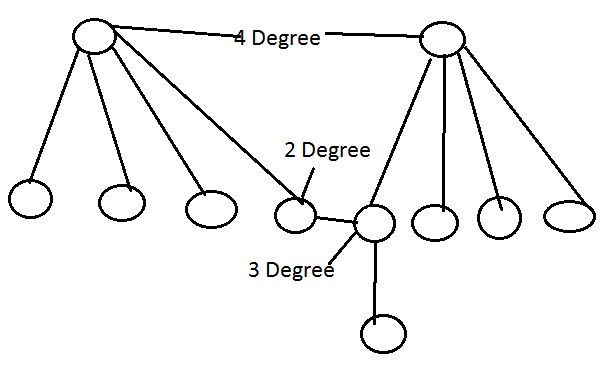# December 2014 - Paper 2

1:

A certain tree has two vertices of degree 4, one vertex of degree 3 and one vertex of degree 2. If the other vertices have degree 1, how many vertices are there in the graph?

 A. 5 B. n-3 C. 20 D. 11 Answer Report Discuss Option: D Explanation :So count of all vertices is 11                  OR A tree of n vertices have n-1 edges.  the sum of all the degree in a graph is equal to twice the no of edge Hence 2*4+1*3+1*2+(n-4)*1=2(n-1) 13+n-4=2n-2 hence n=11. Click on Discuss to view users comments. Write your comments here:
2:

The size of the ROM required to build an 8-bit adder/subtractor with mode control, carry input, carry output and two's complement overflow output is given as

 A. 216 x 8 B. 218 x 10 C. 216 x 10 D. 218 x 8 Answer Report Discuss Option: B Explanation : Click on Discuss to view users comments. Write your comments here:
3:

The best normal form of relation scheme R(A. B, C, D) along with the set of functional dependencies F = {AB → C, AB → D, C → A, D → B} is

 A. Boyce-Codd Normal form B. Third Normal form C. Second Normal form D. First Normal form Answer Report Discuss Option: C Explanation : Click on Discuss to view users comments. Write your comments here:
4:

In a two-pass assembler, symbol table is

 A. Generated in first pass B. Generated in second pass C. Not generated at all D. Generated and used only in second pass Answer Report Discuss Option: C Explanation : Click on Discuss to view users comments. Write your comments here:
5:

Match the following :

List - I                                List - II

a. Call control protocol         i. Interface between Base Transceiver Station (BTS) and Base Station Controller (BSC)